# Computing field values

Several mechanisms will be provided in TROEPS for computing field values: constraints, default inferences and tasks.

## Implicit versus hypothetical computation

Not all the information about the objects is explicitly provided. In fact, some slot can remain unfilled (or undefined). TROEPS provides mechanisms for computing the values of these fields. However, they are not all at the same level because leaving a field unfilled can have several meanings:
• It can mean that the field has no value for the object;
• It can mean that the field value is unknown to the users;
• It can mean that the field value is known to the users to be the value that TROEPS will compute and thus the users do not care about filling it.
These interpretation are different but usually, knowledge representation systems do not distinguish them. In TROEPS, there are computation mechanisms for the second and the third item and they are clearly distinguished. Concerning the second meaning, since the users do not know the value, the system will only be able to compute hypothetical values, i.e. guesses based on the particular position of the object in the taxonomies. Concerning the third meaning, TROEPS is able to compute an implicit value which is the true value of the object (provided that the user has described the methods for computing the value). For instance, imagine that, in the knowledge base presented above, it is considered that if no value is given to the field second-hand, this means that the corresponding house is second hand (and thus the field value is true). This can be used at input time in order to fill only the houses which are brand new ones (implicit value) or this can be used when the status of the house is unknown that it is a second hand house (hypothetical value, which seems statistically reasonable since there are more second hand houses to sell). These are two different interpretation which, in turn, induce totally different behaviour from the users of the knowledge base.
There is a third kind of computing mechanisms called constraints which are not available in the present version of TROEPS.

These are not the only differences between hypothetical and implicit computations. As usual, the implicit computations are part of the "ontological aspect" of the objects and thus are declared in the concept. As a matter of fact, since these implicit computations return the true value of the field, this value does not depend on the classes the object is attached to. The user can declare several methods in order to compute the value but they are supposed to all return the same value and thus only one value is returned by the system. Lastly, since the value is the true value, it is available by using the tr-get-value primitive.
Hypothetical values are guesses depending on the position of the object in the taxonomies. Thus, the inference mechanisms are declared in the classes. For the same object, they can return different values which are concurrent guesses. The hypothetical values are computed on demand through the tr-guess-value primitive. Since, there can be several hypothetical values (at most one per viewpoint), tr-guess-value returns a list of values (ordered in the same way the viewpoints are ordered in the call). If no hypothetical value has been found, it returns the undefined value.

Each mechanism available in TROEPS is described below. There exists only one kind of implicit computation called "procedural detachment" and which, in the current version of TROEPS is mere function call. There exist four kinds of hypothesising mechanisms: default values, value passing, procedural detachment (again, declared in classes) and filtering. These mechanisms are parallel to four exact value computation mechanisms available in TROEPS or other systems:

 Mechanism TROEPS exact computation SHIRKA equivalent Default value Type reduced to a singleton ( \$value in SHIRKA) \$default Value passing Constraint \$var<- Procedural detachment Task invocation \$sib-exec Filters Filtering \$sib-filtre

## Hypothetical computation, inheritance by default and ordering

The mechanisms for guessing hypothetical values are inherited by default in the taxonomy. This means that TROEPS will try to obtain the guesses from all the classes the object is attached to. If no mechanism attached to one of these classes returns a value, it will try again with their super-classes and so on so forth. However, as soon as a value is returned, the process will stop with that value (for the taxonomy). If other mechanisms are defined in super-classes they are shadowed by those defined in more specific classes (it is supposed that if they are more specific they are able to provide more adapted guesses).
The computation of hypothetical values is not easy to follow and is thus provided here. However, it is not necessary to understand it for using hypothetical computations. The algorithm is roughly the following:
• When tr-guess-value is called with several viewpoints as arguments it first considers independently each viewpoint in the same order.
• In each taxonomy it tries to find a value by investigating the successive super-classes of the one the object is attached to. If a value is obtained in a class, this value is returned and no other class is considered.
• In each class it tries each inference descriptor in the following order: assign, filters, methods and default. If a value is obtained by a descriptor, this value is returned and no other descriptor is considered.
• In each descriptor there can be several method (but for default) and the system tries each one in the order in which they are stored in the descriptor. The obtained values are checked if they satisfy the particular constraints applying to the object. If a value is obtained by a method and satisfy the constraints, this value is returned and no other method is considered.
• For each method, the constraints of filters and the arguments of procedural detachment must be computed before triggering them. This can require additional hypothetical computation which is triggered exclusively in the current viewpoint. If this computation fails to return a value, then the method fails.
As a result, tr-guess-value returns at most one value by viewpoint.

### Interface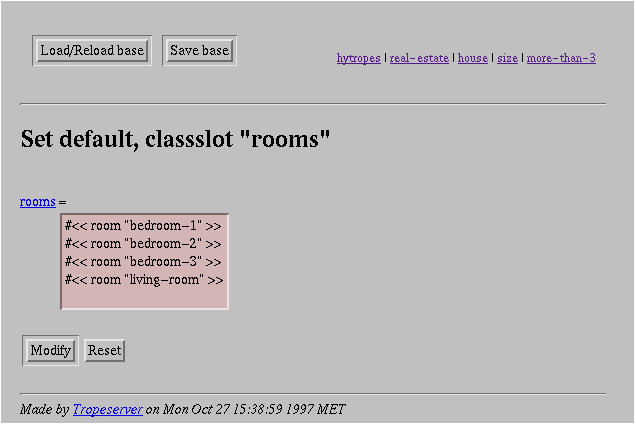Screendump 40: Class field default value modification.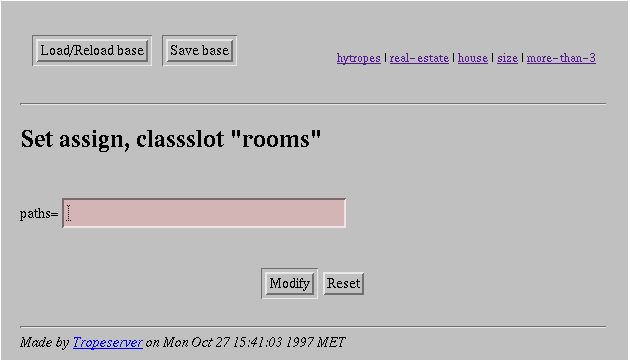Screendump 41: Class field assign modification.

NIY

Screendump 42: Class field filter modification.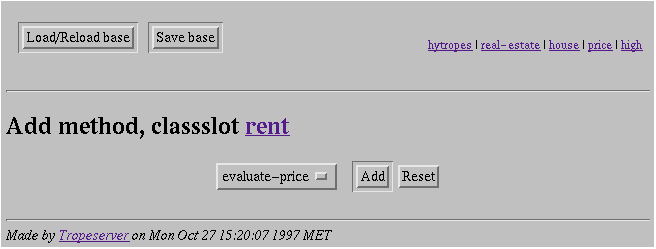Screendump 43: Class field method modification.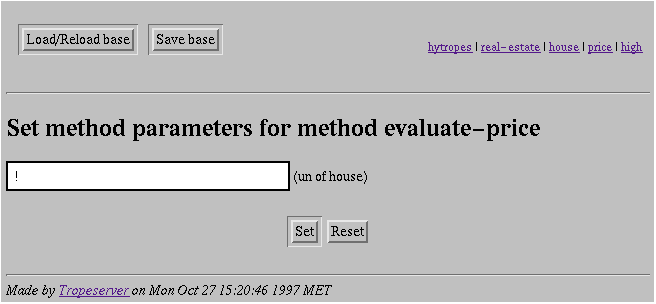Screendump 44: Class field method argument modification.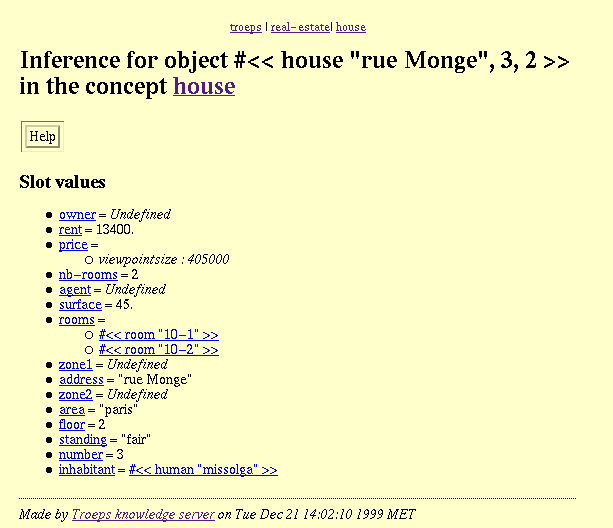Screendump 45: Guessed value display.

### API

(tr-guess-value object slotname rest: views ) function in [libtrp]

-> {(conceptview . value)*}, object object nao, slotname string nsa, ukks , views { conceptview nakv* } ivsp
If the field of name slotname of object object has a value, this value is returned. Otherwise, uses the inference methods stored in the method descriptors of the different classes (under the considered viewpoints) for returning the hypothetical values under each viewpoint.

## Default value

The more basic inference means consists in using a default value for a particular field. The default value is provided in classes and remains valid for the sub-classes as far as no other default value is provided. The example above give the default value second-hand as true for all the houses. However, if a new area is known to have been just recently constructed, then the objects attached to the class corresponding to that area will have the false default value for the field. Then, if an objects is attached to that class the guessed value will be false.
The default classslot descriptor allows to provide a default value for a field which must be an acceptable value for the class.

### API

(tr-get-classslot-default classslot) function in [libtrp]

-> value, classslot classslot nacs
Returns the default value defined and applicable to the class field classslot.
(tr-set-classslot-default classslot value ) function in [libtrp]

-> boolean, classslot classslot nacs, value entity ivsv, kcv
Sets the default value applicable to the class field classslot.
(tr-unset-classslot-default classslot) function in [libtrp]

-> boolean, classslot classslot nacs
Removes the default value applicable to the class field classslot.

### Examples

```? (tr-get-value (tr-object (tr-concept "house") `("rue Monge" 2 3)) "standing")
= #s(<undef>)
? (tr-guess-value (tr-object (tr-concept "house") `("rue Monge" 2 3)) "standing"
(tr-concepview (tr-concept "house") "price"))
= #s(<undef>)
? (tr-set-classslot-default
(tr-classslot (tr-conceptview-root (tr-concepview (tr-concept "house") "price")) "standing")
"high")
= (((tr-conceptview (tr-concept "house") "price") . #s(<undef>)))
? (tr-get-classslot-default
(tr-classslot (tr-conceptview-root (tr-concepview (tr-concept "house") "price")) "standing"))
= "high"
? (tr-guess-value (tr-object (tr-concept "house") `("rue Monge" 2 3)) "standing"
(tr-concepview (tr-concept "house") "price"))
= (((tr-conceptview (tr-concept "house") "price") . "high"))
? (tr-guess-value (tr-object (tr-concept "house") `("rue Monge" 2 3)) "standing"
(tr-concepview (tr-concept "house") "localisation"))
= (((tr-conceptview (tr-concept "house") "localisation") . #s(<undef>)))
? (tr-set-classslot-default
(tr-classslot
(tr-is-in-under (tr-object (tr-concept "house") `("rue Monge" 2 3))
(tr-concepview (tr-concept "house") "localisation"))
"standing")
"low")
= "low"
? (tr-guess-value (tr-object (tr-concept "house") `("rue Monge" 2 3)) "standing")
= (((tr-conceptview (tr-concept "house") "test") . #s(<undef>))
((tr-conceptview (tr-concept "house") "localisation"). "low")
((tr-conceptview (tr-concept "house") "size") . #s(<undef>))
((tr-conceptview (tr-concept "house") "price") . "high"))
? (tr-guess-value (tr-object (tr-concept "house") `("rue Monge" 2 3)) "standing"
(tr-concepview (tr-concept "house") "price"))
= (((tr-conceptview (tr-concept "house") "price") . "high"))
? (tr-unset-classslot-default
(tr-classslot (tr-conceptview-root (tr-conceptview (tr-concept "house") "localisation")) "standing"))
= #s(<undef>)
? (tr-set-classslot-default
(tr-classslot (tr-conceptview-root (tr-concepview (tr-concept "house") "localisation")) "standing")
"high")
= "high"
? (tr-guess-value (tr-object (tr-concept "house") `("rue Monge" 2 3)) "standing")
= (((tr-conceptview (tr-concept "house") "test") . #s(<undef>))
((tr-conceptview (tr-concept "house") "localisation") . "high")
((tr-conceptview (tr-concept "house") "size") . #s(<undef>))
((tr-conceptview (tr-concept "house") "price") . "high"))```

## Paths and slot value passing

Another inference mechanism consists in looking for a plausible value in another field of the same object, or a field of another object which is referred to by the former one. As an example, it could be plausible that someone has the same name as his wife. So the name field can be looked for in the name field of his wife.
This is achieved by specifying a path from the object to where the value can be found. This path is the succession of fields to be used in order to access the value. In the example above, the path is expressed by !.wife in which " !" stands for the object itself and wife is the name of a field. This can be iterated such that !.wife.sons are the sons of the wife and !.wife.sons.wife are the wives of the sons of the wife (daughters in law).
The definition of paths can lead to strange values. For instance, if one tries to define the set of grand-sons by the path !.children.sons, the result should be a set of set of persons (one set per child). It should be better to return just a set of persons (because this is a valid slot value). This is possible through the ":" construct !:children.sons returns the union of the children of the sons of the person.
Now, the assign classslot descriptor allows to provide a value passing specification for a field. The values in the assign descriptor are valid paths from the current class (i.e. paths which can be followed from the instances of the class and which lead to a value of the correct type).
When looking for an hypothetical value for a field, if the assign descriptor contain paths, the paths are followed (in the order of the descriptor content) and the first one to return a value will be considered as the guess. It is noteworthy that there are several paths because a path can fail to return a value if one of the elements along the path is missing.

### API

(tr-make-path conceptslots) function in [libtrp]

-> path, conceptslots {conceptslot*} nacs
Creates and returns a path starting at the concept of the first element of conceptslots and following the sequence of these conceptslots.
(tr-path-p anything) function in [libtrp]

-> boolean, anything entity
Returns anything if the argument anything is a path, () otherwise.
( tr-get-classslot-assigns classslot) function in [libtrp]

-> {path*}, classslot classslot nacs
Returns the set of path assignments defined and applicable to the class field classslot.
(tr-add-classslot-assign classslot paths ) function in [libtrp]

-> boolean, classslot classslot nacs, paths ( path* )
Adds a path to those defined and applicable to the class field classslot.
(tr-rem-classslot-assign classslot paths ) function in [libtrp]

-> boolean, classslot classslot nacs, paths ( path* )
Removes a path from those applicable to the class field classslot.

### Examples

```? (tr-get-value (tr-object (tr-concept "house") `("rue Monge" 2 3)) "inhabitant")
= #s(<undef>)
? (tr-guess-value (tr-object (tr-concept "house") `("rue Monge" 2 3)) "inhabitant"
(tr-concepview (tr-concept "house") "size"))
= (((tr-conceptview (tr-concept "house") "size") . #s(<undef>)))
? (tr-add-classslot-assign (tr-classslot (tr-conceptview-root (tr-conceptview (tr-concept "house") "size")) "inhabitant") (tr-make-path (tr-concept "house") "!.owner"))
= ((tr-make-path (tr-concept "house") "!.owner"))
? (tr-get-classslot-assign (tr-classslot (tr-conceptview-root (tr-conceptview (tr-concept "house") "size")) "inhabitant")
= ((tr-make-path (tr-concept "house") "!.owner"))
? (tr-path-p (car (tr-get-classslot-assign (tr-classslot (tr-conceptview-root (tr-conceptview (tr-concept "house") "size")) "inhabitant"))
= ((tr-make-path (tr-concept "house") "!.owner"))
? (tr-set-value (tr-object (tr-concept "house") '("rue Monge" 2 3))
"owner"
(tr-create-object (tr-concept "human") '("M.M")))
= (tr-object (tr-concept "human") '("M.M"))
? (tr-guess-value (tr-object (tr-concept "house") `("rue Monge" 2 3)) "inhabitant")
= (((tr-conceptview (tr-concept "house") "test") . #s(<undef>))
((tr-conceptview (tr-concept "house") "localisation")
(tr-object (tr-concept "human") '("M.M")))
((tr-conceptview (tr-concept "house") "price") . #s(<undef>)))
? (tr-rem-classslot-assign (tr-classslot (tr-conceptview-root (tr-conceptview (tr-concept "house") "size")) "inhabitant") (car (tr-get-classslot-assign (tr-classslot (tr-conceptview-root (tr-conceptview (tr-concept "house") "size")) "inhabitant"))))
= ()
? (tr-guess-value (tr-object (tr-concept "house") `("rue Monge" 2 3)) "inhabitant"))
= (((tr-conceptview (tr-concept "house") "test") . #s(<undef>))
((tr-conceptview (tr-concept "house") "localisation"). #s(<undef>))
((tr-conceptview (tr-concept "house") "price") . #s(<undef>)))```

## Computation by filter

The computation by filters can be used by set valued slots. It consists in creating a filter which returns the set of filtered values which will then be returned as the guessed value for the field. These filters can support additional constraints provided through the usual filter and class manipulation primitives. Moreover, these constraints can be computed by inference descriptors which are defined with regard to a current object.
The filters classslot descriptor allows to provide filters for computing a field.
When a value is required and a filter computation is necessary, first the actual constraints of the filter are computed with regard to the current object and then the filtered object are obtained as usual (see the "Filters" section in the previous part of the manual).

### API

(tr-get-classslot-filters classslot) function in [libtrp]

-> { filter* }, classslot classslot nacs
Returns the set of filters defined for and applicable to the class field classslot.
(tr-add-classslot-filters classslot filters ) function in [libtrp]

-> boolean, classslot classslot nacs, filters ( filter* ), adf
Add a new filter to the set of filters defined for and applicable to the class field classslot.
(tr-rem-classslot-filters classslot filters ) function in [libtrp]

-> boolean, classslot classslot nacs, filters ( filter* ), adf
Removes a filter from the set of filters defined for and applicable to the class field classslot.

### Example

```? (tr-get-value (tr-object (tr-concept "house") `("rue Monge" 2 3)) "rooms")
= #s(<undef>)
? (tr-guess-value (tr-object (tr-concept "house") `("rue Monge" 2 3)) "rooms"
(tr-concepview (tr-concept "house") "size"))
= (((tr-conceptview (tr-concept "house") "size") . #s(<undef>)))
(tr-classslot
(tr-conceptview-root (tr-conceptview (tr-concept "house") "size")) "rooms")
(tr-create-filter (tr-concept "room")))
= (tr-filter (tr-concept "room"))
? (tr-get-classslot-filters
(tr-classslot
(tr-conceptview-root (tr-conceptview (tr-concept "house") "size")) "rooms"))
= ((tr-filter (tr-concept "room")))
? (tr-create-complete-object (tr-concept "room") ()
(list (cons "id" "rm12")
(cons "appart" (tr-object (tr-concept "house") `("rue Monge" 2 3)))))
= (tr-object (tr-concept "room") `("rm12"))
? (tr-create-complete-object (tr-concept "room") ()
(list (cons "id" "rm13")
(cons "appart" (tr-object (tr-concept "house") `("rue Monge" 2 3)))))
= (tr-object (tr-concept "room") `("rm13"))
? (tr-create-complete-object (tr-concept "room") ()
(list (cons "id" "rm14")
(cons "appart" (tr-object (tr-concept "house") `("rue Monge" 2 3)))))
= (tr-object (tr-concept "room") `("rm14"))
? (tr-rem-classslot-filter
(tr-classslot
(tr-conceptview-root (tr-conceptview (tr-concept "house") "size")) "rooms")
(car (tr-get-classslot-filter
(tr-classslot
(tr-conceptview-root (tr-conceptview (tr-concept "house") "size")) "rooms"))))
= ()
? (tr-guess-value (tr-object (tr-concept "house") `("rue Monge" 2 3)) "rooms")
= (((tr-conceptview (tr-concept "house") "test") . #s(<undef>))
((tr-conceptview (tr-concept "house") "localisation"). #s(<undef>))
((tr-conceptview (tr-concept "house") "price") . #s(<undef>)))```

Task or method invocation covers what has been called procedural detachment and the use of a function in order to compute a value. The tasks are actually simple (C or talk) functions invoked on their arguments and returning a value. They are described through the generic term " method ".
The methods classslot or conceptslot descriptor allows to provide a task specification for a field. These specification follows the same line, they consists in a list beginning by the method name (the name of the function to be called) and the list of its argument specification (in the correct order). These arguments can be either concrete values or paths.
The tasks are computed in the order under which they are in the descriptor. The computation stops with the first succeeding call. Before computing the task, the paths taken as arguments are evaluated. If any of them return no value then the task invocation fails. Once the arguments are computed, the function is called against them. It returns either undefined ( (unbound-slot-value)) or another value. In the first event, the task fails, in the second one, the value is returned.
Before they can be used, the methods must be declared with their signature. This allows static type checking of the method when it is put in the field. The concepts involved in the method type do not have to be declared when the method is declared (so this can be done before loading the base for instance) but they must have been loaded when it is added to a field.

### API

(tr-declare-method name args result ) function in [libtrp]

-> symbol, name symbol aem, arg s ((string . string)*) naks , result (string . string) naks
Declares a mehod of name name and signature args -> result. The signature is made of couple constructor/type as for defining a conceptslot.
(tr-get-conceptslot-methods conceptslot) function in [libtrp]

-> ((symbol (entity|path)* )*), conceptslot conceptslot naks
Returns the set of methods attached to the concept field conceptslot.
(tr-add-conceptslot-method conceptslot method ) function in [libtrp]

-> boolean, conceptslot conceptslot naks, method (symbol nas (entity|path)* ) ivm
Adds the new inference methods methods for the concept field conceptslot. The method specification method is a list begining by the method name followed by the method arguments. The arguments are either values or paths. They return either a value for the corresponding field of the object, either the result of the function (unbound-slot-value). When necessary, these methods are evaluated from the first to the last in this list.
(tr-rem-conceptslot-method conceptslot method ) function in [libtrp]

-> boolean, conceptslot conceptslot naks, method (symbol nas (entity|path)* ) ivm
Removes the method method from those attached to the concept field conceptslot.
(tr-get-classslot-methods classslot) function in [libtrp]

-> ((symbol (entity|path)* )*), classslot classslot nacs
Returns the set of methods defined for and applicable to the class field classslot.
(tr-add-classslot-method classslot method ) function in [libtrp]

-> boolean, classslot classslot nacs, methods ((symbol nas (entity|path)* ) ivm )*
Sets the new inference methods methods for the class field classslot. The method specification method is a list begining by the method name followed by the method arguments. The arguments are either values or paths. They return either a value for the corresponding field of the object, either the result of the function (unbound-slot-value). When necessary, these methods are evaluated from the first to the last in this list.
(tr-rem-classslot-method classslot method ) function in [libtrp]

-> boolean, classslot classslot nacs, , methods ((symbol nas (entity|path)* ) ivm )*
Removes the inference methods methods for the class field classslot.

### Example

```? (tr-get-value (tr-object (tr-concept "house") `("rue Monge" 2 3)) "price")
= #s(<undef>)
? (tr-guess-value (tr-object (tr-concept "house") `("rue Monge" 2 3)) "price"
(tr-concepview (tr-concept "house") "size"))
= (((tr-conceptview (tr-concept "house") "size") . #s(<undef>)))
? (defun evaluate-price (house)
(if (undefinedp (tr-get-value house "surface"))
(unbound-slot-value)
(let ((basis (* (tr-get-value house "surface") 9000)))
(cond
((tr-superclass-p
(tr-class (tr-conceptview (tr-concept "house") "size") "more-than-3")
(tr-is-in-under house (tr-conceptview (tr-concept "house") "size")))
(int (* basis 0.9)))
((tr-superclass-p
(tr-class (tr-conceptview (tr-concept "house") "size") "more-than-3")
(tr-is-in-under house (tr-conceptview (tr-concept "house") "size")))
(int (* basis 1.2)))
(t (int basis))))))
= evaluate-price
? (tr-declare-method 'evaluate-price
(list (cons "un" "house"))
(cons "un" "entier"))
= evaluate-price
(tr-classslot (tr-conceptview-root
(tr-conceptview (tr-concept "house") "size"))
"price")
(list 'evaluate-price (tr-make-path (tr-concept "house") "!")))
= ((evaluate-price (tr-make-path (tr-concept "house") "!")))
? (tr-get-classslot-methods
(tr-classslot
(tr-conceptview-root (tr-conceptview (tr-concept "house") "size"))
"price"))
= ((evaluate-price (tr-make-path (tr-concept "house") "!")))
? (tr-guess-value (tr-object (tr-concept "house") '("rue Monge" 2 3)) "price")
= (((tr-conceptview (tr-concept "house") "test") . #s(<undef>))
((tr-conceptview (tr-concept "house") "size") . #s(<undef>))
((tr-conceptview (tr-concept "house") "localisation") . #s(<undef>))
((tr-conceptview (tr-concept "house") "price") . #s(<undef>)))
? (tr-set-value (tr-object (tr-concept "house") '("rue Monge" 2 3)) "surface" 45.)
= 45.
? (tr-guess-value (tr-object (tr-concept "house") '("rue Monge" 2 3)) "price")
= (((tr-conceptview (tr-concept "house") "test") . #s(<undef>))
((tr-conceptview (tr-concept "house") "size") . 405000)
((tr-conceptview (tr-concept "house") "localisation") . #s(<undef>))
((tr-conceptview (tr-concept "house") "price") . #s(<undef>)))
? (tr-rem-classslot-method
(tr-classslot
(tr-conceptview-root (tr-conceptview (tr-concept "house") "size"))
"price")
(car (tr-get-classslot-methods
(tr-classslot
(tr-conceptview-root (tr-conceptview (tr-concept "house") "size"))
"price"))))
= ()
? (tr-guess-value (tr-object (tr-concept "house") '("rue Monge" 2 3)) "price"))
= (((tr-conceptview (tr-concept "house") "test") . #s(<undef>))
((tr-conceptview (tr-concept "house") "localisation") . #s(<undef>))
((tr-conceptview (tr-concept "house") "size") . #s(<undef>))
((tr-conceptview (tr-concept "house") "price") . #s(<undef>)))
? (tr-get-value (tr-object (tr-concept "house") `("rue Monge" 2 3)) "nb-rooms")
= #s(<undef>)
? (defun re-llen (list)
(if (undefinedp list) (unbound-slot-value) (llen list)))
= re-llen
? (tr-declare-method 're-llen
(list (cons "ensemble" "room"))
(cons "un" "entier"))
= re-llen
(tr-find-conceptslot (tr-find-concept "house") "nb-rooms")
(list 're-llen (tr-make-path (tr-concept "house") "!.rooms")))
= ((re-llen (tr-make-path (tr-concept "house") "!.rooms")))
? (tr-get-conceptslot-methods
(tr-find-conceptslot (tr-find-concept "house") "nb-rooms"))
= ((re-llen (tr-make-path (tr-concept "house") "!.rooms")))
? (tr-get-value (tr-object (tr-concept "house") `("rue Monge" 2 3)) "nb-rooms")
= 2```

## Constraints

While an object-based knowledge representation system like TROEPS is well-suited to describe, organise, infer and store large amounts of knowledge, there is also a need both for reasoning using field domains (and not only field values, like methods do) and for expressing relations (and not only the "part-of" relation) or properties about objects.
In order to achieve these two goals in TROEPS, the constraint paradigm seems to provide a helpful and powerful tool. Indeed, a constraint C(x1,...xn) describes a relation that must be satisfied by a set V = { x1,... xn} of variables (named the constrained variables), each xi having an associated domain Di of values. In usual constraint programming languages, given a set of constraints, a constraint solver applies satisfaction algorithms (to eliminate from each domain, the values that can not appear in any solution) in order to find one, some or all the solutions of this constraint satisfaction problem.
Constraints are not available within this version of TROEPS.

### Constraint constructions

The constraints (or better constraint expressions) are build from constraints predicates (expressing a constraints between several values), constraint operators (expressing the construction of a value from others), paths (designating the variables) and values. For instance:
• = is a constraint predicate (a = b is either true or false),
• * is a constraint operator (constructing an integer from other integers),
• 12 is a value, and
• !.monthly-rent is a path
The expression (* !.monthly-rent 12) is called a constraint term whose operator is * and (= !.yearly-rent (* !.monthly-rent 12)) is called a constraint. It constrains two expressions to be equal.

### Constraints as unary predicates

Typing descriptors reveal themselves inadequate for stating a property the value of an field has to satisfy. Such a property can now be expressed with a unary predicate given by the new descriptor condition.

### API

(tr-make-constraint constraint-predicate rest: constrained-terms)-- function in [libtrp]

-> constraint, constraint-predicate contraint-predicate nacp, constrained-terms ( constrained-term|path|entity * ) nace NIY
Creates and returns a constraint expression representing the application of a constraint predicate against its list of arguments.
(tr-make-constraint-term constraint-operator rest: constrained-terms)-- function in [libtrp]

-> constraint-term, constraint-operator contraint-operator naco, constrained-terms ( constrained-term|path|entity* ) nace NIY
Creates and returns a constraint term representing the application of a constraint operator against its list of arguments.
(tr-concept-add-constraint concept constraint )-- function in [libtrp]

-> boolean, concept concept nak, constraint constraint nact NIY
Adds the constraint constraint to the set of constraints applying to the concept concept.
( tr-concept-rem-constraint concept constraint-exp )-- function in [libtrp]

-> boolean, concept concept nak, constraint constraint nact NIY
Suppresses the constraint constraint from the set of constraints applying to the concept concept.
(tr-concept-constraints concept)-- function in [libtrp]

-> { constraint* }, concept concept nak NIY
Returns the constraints applying to the concept concept.
(tr-class-add-constraint class constraint )-- function in [libtrp]

-> boolean, class class nac, constraint constraint nact NIY
Adds the constraint constraint to the set of constraints applying to the concept concept.
( tr-class-rem-constraint class constraint )-- function in [libtrp]

-> boolean, class class nac, constraint constraint nact NIY
Suppresses the constraint constraint from the set of constraints applying to the concept concept.
(tr-class-constraints class)-- function in [libtrp]

-> { constraint* }, class class nac NIY
Returns the constraints applying to the class class.
(tr-object-add-constraint object constraint)-- function in [libtrp]

-> boolean, object object nao, constraint constraint nact NIY
Adds the constraint expressed by constraint to the set of constraints applying to the object object.
(tr-object-rem-constraint object constraint)-- function in [libtrp]

-> boolean, object object nao, constraint constraint nact NIY
Deletes the constraint constraint to the set of constraints applying to the object object.
(tr-object-constraints object)-- function in [libtrp]

-> { constraint* }, object object nao NIY
Returns the constraints applying to the class class.
(tr-get-classslot-condition classslot)-- function in [libtrp]

-> { constraint-predicate* }, classslot classslot nacs NIY
Returns the conditions of the class field classslot, () if they are not refined in the class field.
(tr-set-classslot-condition classslot condition )-- function in [libtrp]

-> boolean, classslot classslot nacs, condition constraint-predicate nacp NIY
Refines the conditions of the class field classslot by restricting them to the new conditions condition.